Next: Discussion Up: Processing local-stationary data Previous: Implementation and Interface

# Equivalence of filter- and data interpolation

To ensure smoothly space-variant filtering, we overlap neighboring patches, process each patch individually, and interpolate the resulting patch vectors. This patching operation may look ad hoc: Shouldn't we apply a spatially varying operator instead? In the case of a local-stationary filtering operation, the interpolation of filters and the interpolation of data patches approximately yield the same result, if the interpolation weights vary slowly with respect to the filter size. Under such circumstances, the sequence of convolution and interpolation can be commuted. Similar commutations should be permissible for other stationary operators.

I will demonstrate the equivalence of data and filter interpolation on a simple deconvolution example. The local-stationary data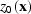has two stationary components. We find a normalized, smoothly varying, weighting function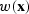that isolates the two stationary components. If we define the deconvolution filters for the two stationary components asand, we can compute two filtered images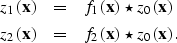(5)
The interpolation of the two filtered images yields the interpolation of the two deconvolved subsets: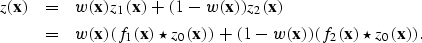(6)

If, however,is slowly varying compared to the size of the filters f1 and f2, the weight function can be assumed to be locally constant. Under this assumption, multiplication and convolution commute. Now, the filter - not the data - is interpolated: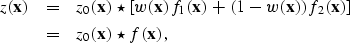(7)
where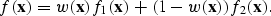In terms of the two scales involved, the filter f varies smoothly on every scale, while the filter components f1 and f2 are locally constant.Next: Discussion Up: Processing local-stationary data Previous: Implementation and Interface
Stanford Exploration Project
3/8/1999